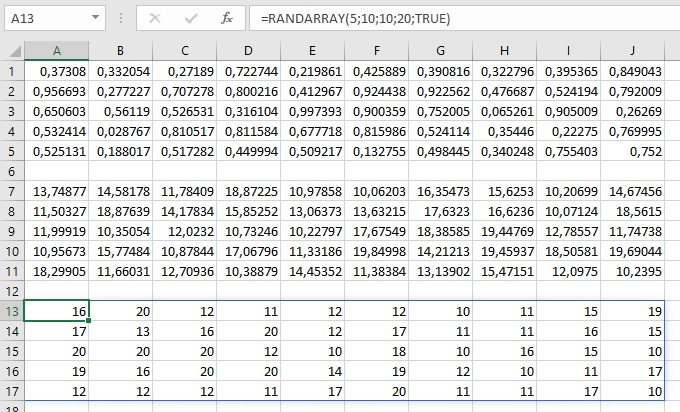# RANDARRAY function

One of the previous “recipes” was about randomly generated numbers, i.e. the use of the RANDBETWEEN function. The RANDARRAY function works similarly to the fact that, unlike the one mentioned above, it generates a random range of numbers (vector or a matrix) based on previously specified rules. This is one of the new Excel functions that is available only to Office 365 subscribers. Let’s see how it is works!

The syntax of this function is simple:

RANDARRAY ([<rows>],[<columns]],[<minimum>],[<maximum>],[<integer>])

And these are the arguments:

• rows – the number of rows that is generated
• columns – the number of columns that are generated
• minimum, maximum – minimum and maximum value. The default minimum is 0 and the maximum is 1
• integer – if the argument has the value TRUE then the function generates integers. The default value is FALSE

For example, if we write the formula:

= RANDARRAY(5,10)

this function will randomly generate a matrix of numbers between 0 and 1 which is 5 rows high and 10 columns wide. if we change the formula in the following way:

= RANDARRAY (5,10,10,20)

we get a matrix of dimensions 5×10 randomly selected decimal numbers between 10 and 20. If we add the last parameter and give it a value of TRUE, it will randomly generate integer numbers between 10 and 20:

= RANDARRAY(5,10,10,20,TRUE)

You can use this feature to easily generate test data.